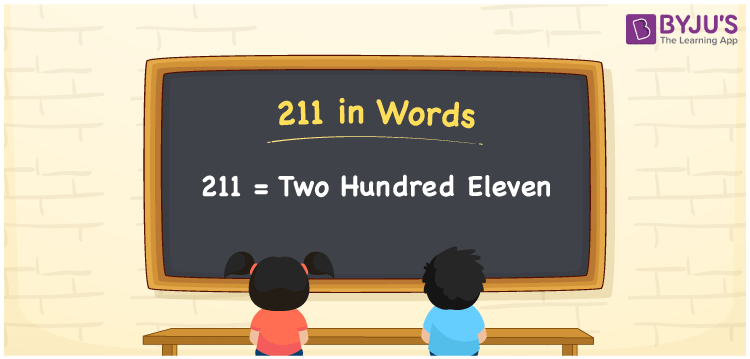# 211 in words

211 in words is written as Two Hundred and Eleven. 211 represents the count or value. The article on Counting Numbers can give you an idea about count or counting. The number 211 is a 3 digit number that is used in expressions related to money, days, distance, length, weight and so on. Let us consider an example for 211. “This street number is Two Hundred and Eleven”.

 211 in words Two Hundred and Eleven Two Hundred and Eleven in Numbers 211

## 211 in English Words## How to Write 211 in Words?

We can convert 211 to words using a place value chart. The number 211 has 3 digits, so let’s make a chart that shows the place value up to 3 digits.

 Hundreds Tens Ones 2 1 1

Thus, we can write the expanded form as:

2 × Hundred + 1 × Ten + 1 × One

= 2 × 100 + 1 × 10 + 1 × 1

= 211

= Two Hundred and Eleven.

211 is the natural number that is succeeded by 210 and preceded by 212.

211 in words – Two Hundred and Eleven.

Is 211 an odd number? – Yes.

Is 211 an even number? – No.

Is 211 a perfect square number? – No.

Is 211 a perfect cube number? – No.

Is 211 a prime number? – Yes.

Is 211 a composite number? – No.

## Solved Example

1. Write the number 211 in expanded form

Solution: 2 × 100 + 1 × 10 + 1 × 1

We can write 211 = 200 + 10 + 1

= 2 × 100 + 1 × 10 + 1 × 1.

## Frequently Asked Questions on 211 in words

Q1

### How to write the number 211 in words?

211 in words is written as Two Hundred and Eleven.
Q2

### Is 211 divisible by 3?

No. 211 is not divisible by 3.
Q3

### Is 211 a prime number?

Yes. 211 is a prime number.